# A chemist titrates 80.0 mL of a 0.3371 M ammonia (NH3) solution with 0.5400 M HCl...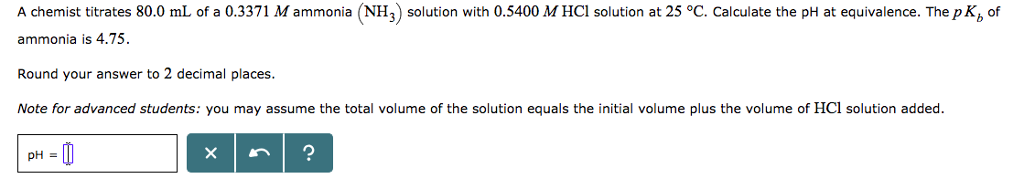A chemist titrates 80.0 mL of a 0.3371 M ammonia (NH3) solution with 0.5400 M HCl solution at 25 °C. Calculate the pH at equivalence. The pK, of ammonia is 4.75. Round your answer to 2 decimal places. Note for advanced students: you may assume the total volume of the solution equals the initial volume plus the volume of HCl solution added.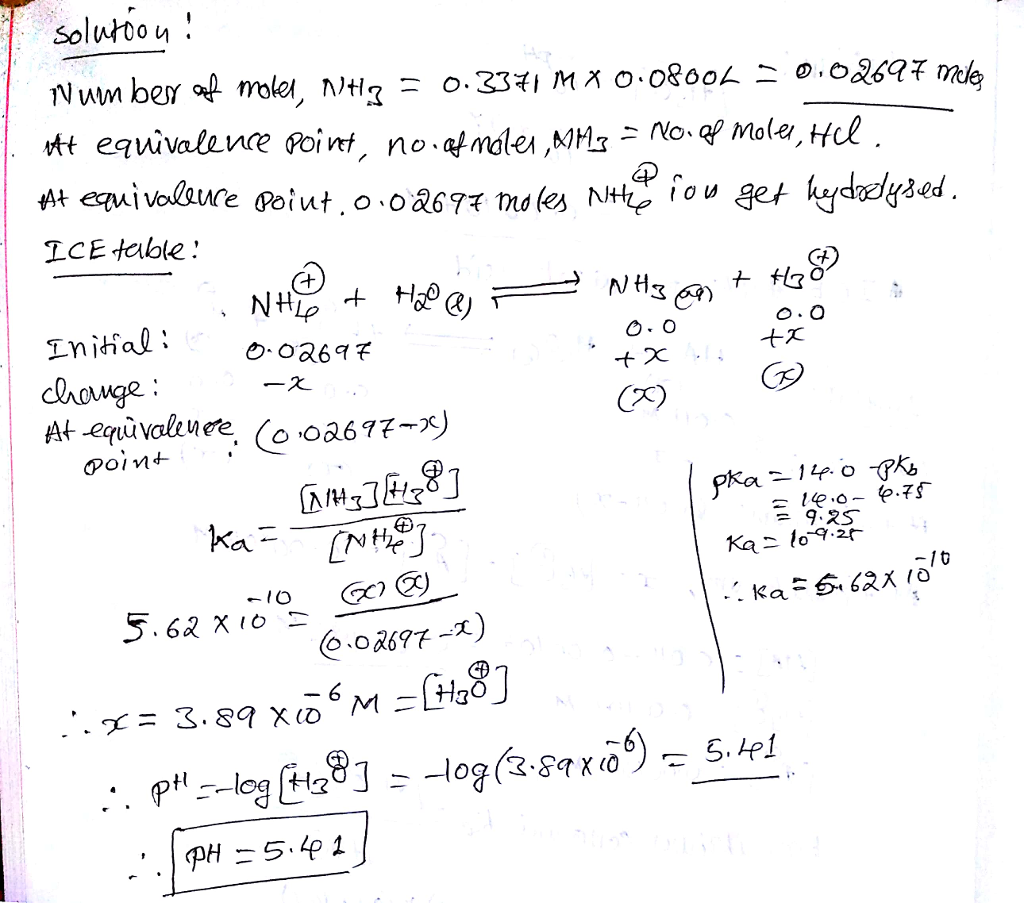#### Earn Coin

Coins can be redeemed for fabulous gifts.

Similar Homework Help Questions
• ### A chemist titrates 100.0 mL of a 0.3204 M ammonia (NH) solution with 0.7322 MHBr solution...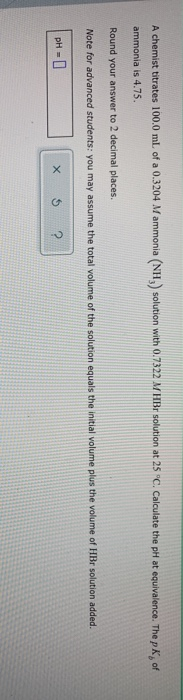A chemist titrates 100.0 mL of a 0.3204 M ammonia (NH) solution with 0.7322 MHBr solution at 25°C. Calculate the pH at equivalence. The pK, of ammonia is 4.75. Round your answer to 2 decimal places. Note for advanced students: you may assume the total volume of the solution equals the initial volume plus the volume of HBr solution added. PHO X 5 ?

• ### A chemist titrates 220.0 mL of a 0.7177 M ethylamine (C2HzNH) solution with 0.5582 M HCl...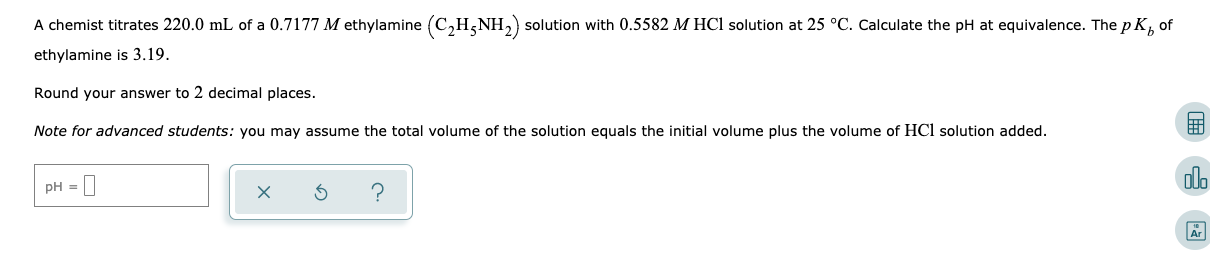A chemist titrates 220.0 mL of a 0.7177 M ethylamine (C2HzNH) solution with 0.5582 M HCl solution at 25 °C. Calculate the pH at equivalence. The pK, of ethylamine is 3.19. Round your answer to 2 decimal places. Note for advanced students: you may assume the total volume of the solution equals the initial volume plus the volume of HCl solution added. pH = 0 1 x 6 ?

• ### An analytical chemist is titrating 227.4mL of a 0.5200M solution of ammonia NH3 with a 0.5400M...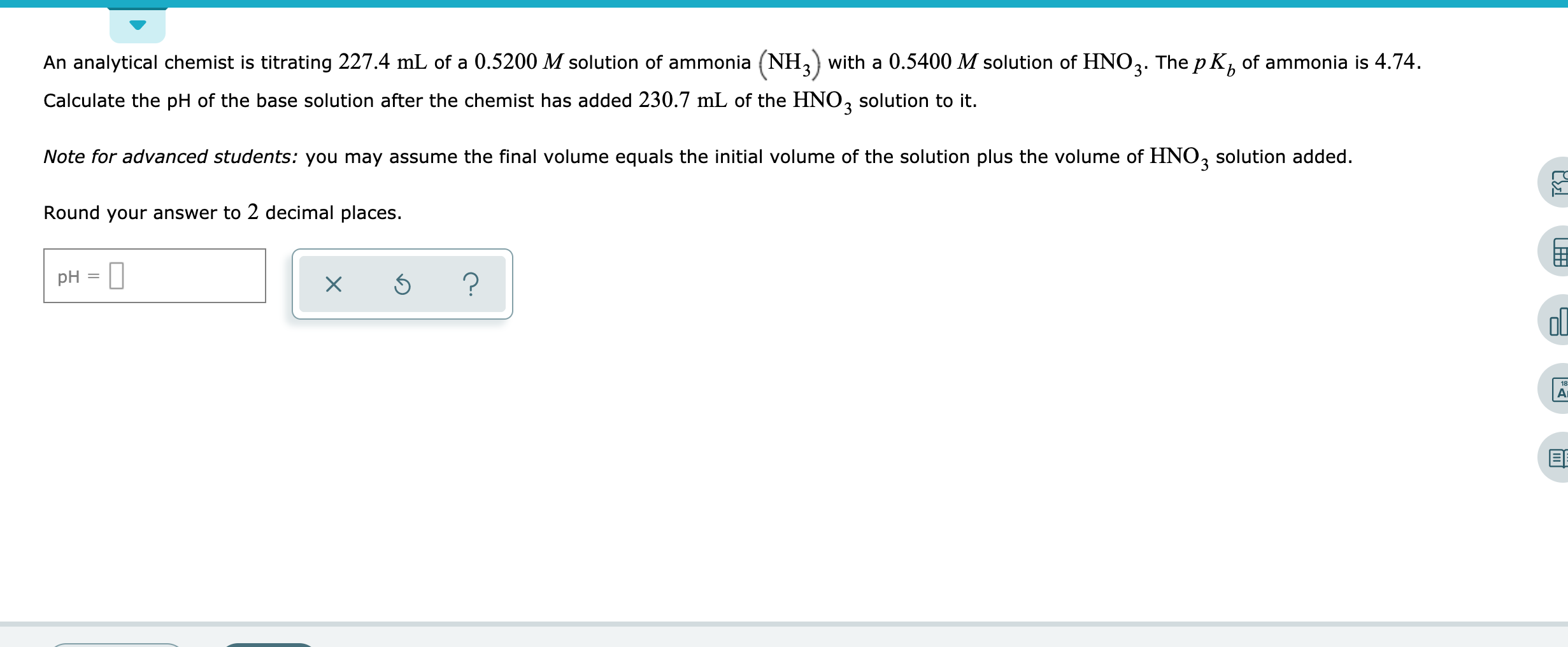An analytical chemist is titrating 227.4mL of a 0.5200M solution of ammonia NH3 with a 0.5400M solution of HNO3. The pKb of ammonia is 4.74. Calculate the pH of the base solution after the chemist has added 230.7mL of the HNO3 solution to it. Note for advanced students: you may assume the final volume equals the initial volume of the solution plus the volume of HNO3solution added. Round your answer to 2 decimal places. An analytical chemist is titrating 227.4...

• ### A chemist titrates 220.0 mL of a 0.4168 M pyridine CHN solution with 0.5811 MHBr solution...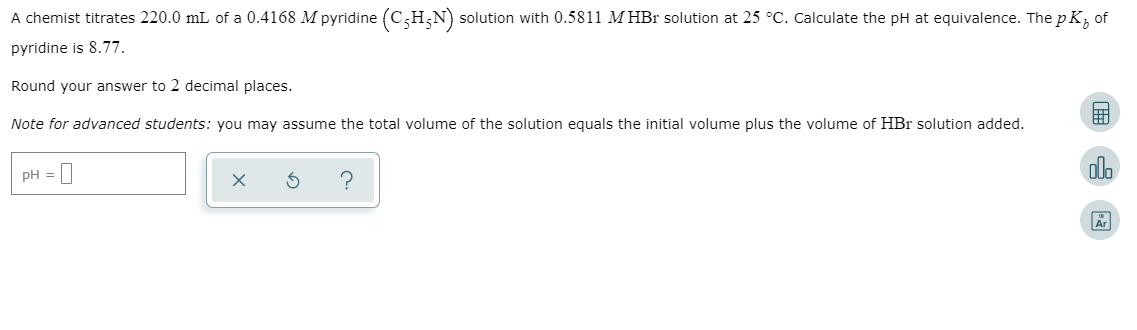A chemist titrates 220.0 mL of a 0.4168 M pyridine CHN solution with 0.5811 MHBr solution at 25 C. Calculate the pH at equivalence. The pK of pyridine is 8.77. Round your answer to 2 decimal places Note for advanced students: you may assume the total volume of the solution equals the initial volume plus the volume of HBr solution added pH ? Ar

• ### An analytical chemist is titrating 149.5 mL of a 0.8000 M solution of ammonia (NH3) with...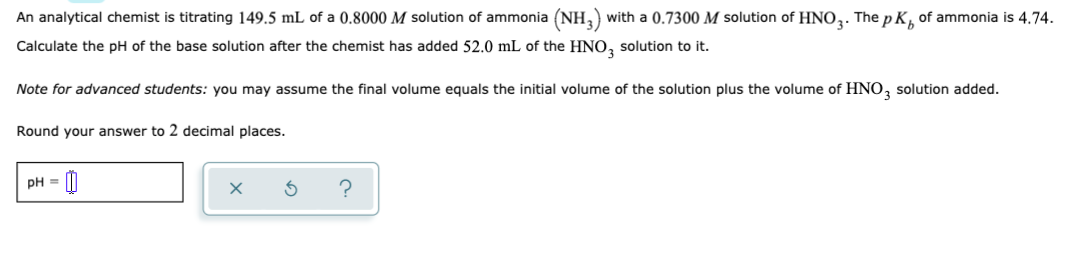An analytical chemist is titrating 149.5 mL of a 0.8000 M solution of ammonia (NH3) with a 0.7300 M solution of HNO3. The pK, of ammonia is 4.74. Calculate the pH of the base solution after the chemist has added 52.0 mL of the HNO3 solution to it. Note for advanced students: you may assume the final volume equals the initial volume of the solution plus the volume of HNO3 solution added. Round your answer to 2 decimal places. pH...

• ### A chemist titrates 150.0 mL of a 0.1029 M potassium hydroxide (KOH) solution with 0.8645 M...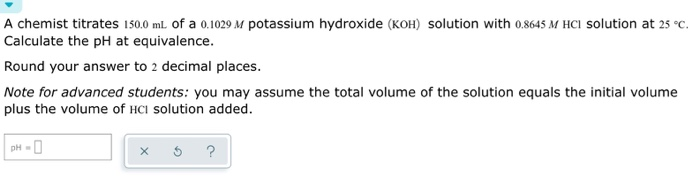A chemist titrates 150.0 mL of a 0.1029 M potassium hydroxide (KOH) solution with 0.8645 M HCl solution at 25°C. Calculate the pH at equivalence. Round your answer to 2 decimal places. Note for advanced students: you may assume the total volume of the solution equals the initial volume plus the volume of HCl solution added. x 5 ?

• ### A chemist titrates 200.0 mL of a 0.7681 M hydrocyanic acid (HCN) solution with 0.5271 M...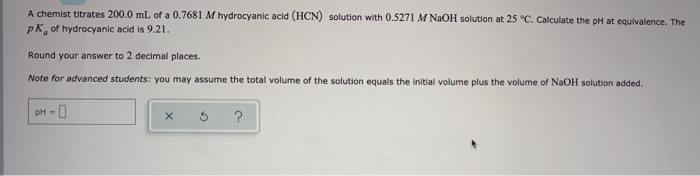A chemist titrates 200.0 mL of a 0.7681 M hydrocyanic acid (HCN) solution with 0.5271 M NaOH solution at 25 °C. Calculate the pH at equivalence. The pk of hydrocyanic acid is 9.21. Round your answer to 2 decimal places. Note for advanced students: you may assume the total volume of the solution equals the initial volume plus the volume of NaOH solution added. pH- х ?

• ### A chemist titrates 140.0 mL of a 0.0952 M aniline (CGH-NH2) solution with 0.7849 M HBr...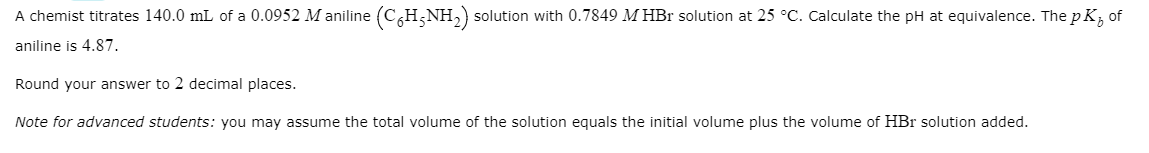A chemist titrates 140.0 mL of a 0.0952 M aniline (CGH-NH2) solution with 0.7849 M HBr solution at 25 °C. Calculate the pH at equivalence. The pK; of aniline is 4.87. Round your answer to 2 decimal places. Note for advanced students: you may assume the total volume of the solution equals the initial volume plus the volume of HBr solution added.

• ### A chemist titrates 160.0 mL of a 0.3337 M carbonic acid (H2CO3) solution with 0.4095 M...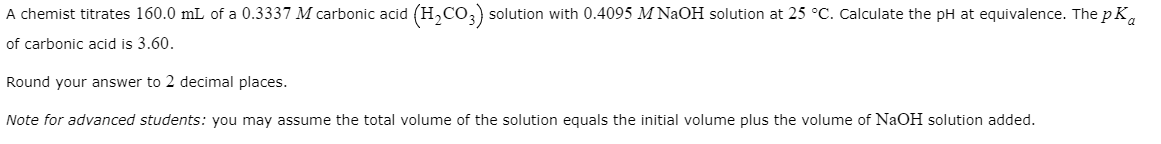A chemist titrates 160.0 mL of a 0.3337 M carbonic acid (H2CO3) solution with 0.4095 M NaOH solution at 25 °C. Calculate the pH at equivalence. The pK, of carbonic acid is 3.60. Round your answer to 2 decimal places. Note for advanced students: you may assume the total volume of the solution equals the initial volume plus the volume of NaOH solution added.

• ### A chemist titrates 50.0 ml. of a 0.6210 Maniline (CH NH) solution with 0.2970 M HNO,...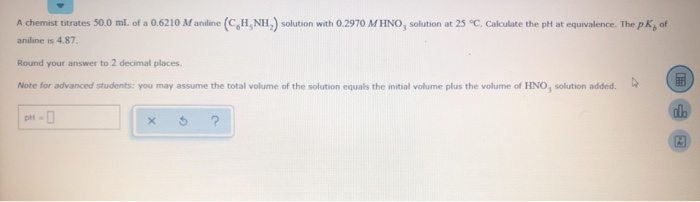A chemist titrates 50.0 ml. of a 0.6210 Maniline (CH NH) solution with 0.2970 M HNO, solution at 25°C. Calculate the ph at equivalence. The pK, of aniline is 4.87 Round your answer to 2 decimal places. Note for advanced students: you may assume the total volume of the solution equals the initial volume plus the volume of HNO, solution added. pH- x 5 ?